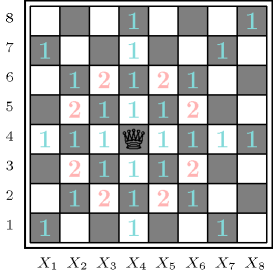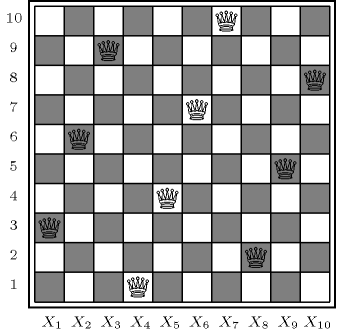### 3.7.163. n-Amazons

modelling: n-Amazons A constraint that can be used for modelling the $n$-Amazons problem. Place $n$ Amazons on an $n$ by $n$ chessboard in such a way that no Amazon attacks another. We say that two columns (respectively two rows) of a chessboard are almost adjacent if and only if the two columns (respectively the two rows) are separated by a single column (respectively a single row). Two Amazons attack each other if at least one of the following conditions holds:

1. They are located on the same column, on the same row or on the same diagonal.

As shown by these conditions, an Amazon combines the movements of a queen and of a knight. Figure 3.7.43 illustrates the movements of an Amazon. The $n$-Amazons problem has no solution when $n$ is smaller than 10.

##### Figure 3.7.43. Illustration of the moves of an Amazon: moves labelled by 1 correspond to queen's moves, while moves labelled by 2 correspond to knight's movesWe now show how to model the $n$-Amazons problem with six global constraints. We start from the model that is used for the n-queens problem. We associate to the ${i}^{th}$ column of the chessboard a domain variable ${X}_{i}$ that gives the row number where the corresponding queen is located.

If we combine the constraints of the form $|{X}_{i}-{X}_{i+1}|\ne 2$ $\left(1\le i\le n-1\right)$ with the three $\mathrm{𝚊𝚕𝚕𝚍𝚒𝚏𝚏𝚎𝚛𝚎𝚗𝚝}$ constraints we get the conjunction of constraints ${X}_{i}-{X}_{i+1}\ne 0$ $\wedge$ $|{X}_{i}-{X}_{i+1}|\ne 1$ $\wedge$ $|{X}_{i}-{X}_{i+1}|\ne 2$ $\left(1\le i\le n-1\right)$. This conjunction of three disequalities can be expressed as a single inequality of the form $|{X}_{i}-{X}_{i+1}|>2$ $\left(1\le i\le n-1\right)$. Furthermore all these inequalities can be combined into a single $\mathrm{𝚜𝚖𝚘𝚘𝚝𝚑}$ constraint of the form $\mathrm{𝚜𝚖𝚘𝚘𝚝𝚑}$$\left(n-1,2,〈{X}_{1},{X}_{2},\cdots ,{X}_{n}〉\right)$.Since we enforce for all pairs of consecutive variables ${X}_{i}$, ${X}_{i+1}$ $\left(1\le i\le n-1\right)$ the constraint $|{X}_{i}-{X}_{i+1}|>2$, the name $\mathrm{𝚜𝚖𝚘𝚘𝚝𝚑}$ seems odd. However the name $\mathrm{𝚜𝚖𝚘𝚘𝚝𝚑}$ stands from the situation where the number of inequalities constraints should be minimised. Similarly we get the constraints $|{X}_{2·i+1}-{X}_{2·i+3}|>2$ $\left(0\le i\le ⌊\frac{n-3}{2}⌋\right)$ and $|{X}_{2·i}-{X}_{2·i+2}|>2$ $\left(1\le i\le ⌊\frac{n-2}{2}⌋\right)$. Again we obtain two $\mathrm{𝚜𝚖𝚘𝚘𝚝𝚑}$ constraints of the form $\mathrm{𝚜𝚖𝚘𝚘𝚝𝚑}$$\left(⌊\frac{n-1}{2}⌋,2,〈{X}_{1},{X}_{3},\cdots ,{X}_{n-1+n\mathrm{mod}2}〉\right)$ and $\mathrm{𝚜𝚖𝚘𝚘𝚝𝚑}$$\left(⌊\frac{n-2}{2}⌋,2,〈{X}_{2},{X}_{4},\cdots ,{X}_{n-n\mathrm{mod}2}〉\right)$.

Finally, the $\mathrm{𝚒𝚗𝚟𝚎𝚛𝚜𝚎}$ constraint can also be used as a channelling constraint if we want to create an additional variable for each row. This may be for instance the case if we want to have a heuristics for selecting first the column or the row that has the smallest number of possibilities.

##### Figure 3.7.44. The unique solution to the 10-Amazons problem (modulo symmetries)Figure 3.7.44 shows the unique solution, modulo symmetries, to the $n$-Amazons problem for $n=10$. We have the following conjunction of constraints: